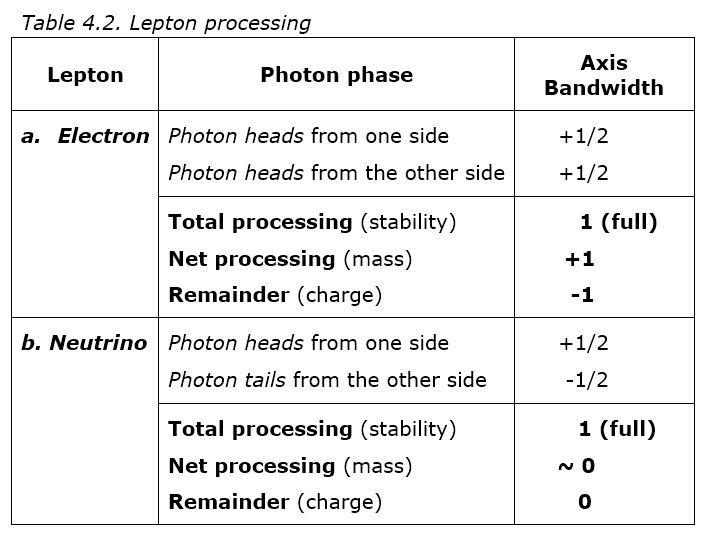# QR4.3.3 The Neutrino Byproduct

The processing that explains matter also explains charge but what about the electron’s brother neutrino? Electrons are critical to our world, as without them there is no chemistry and no life, but our universe also contains a “little nothing” that until recently we didn’t even know existed – the neutrino. The sun floods the earth with vast numbers of them each day but they mostly pass through us like ghosts. Neutrinos seem quite pointless, so why did nature make so many of them?

The standard model expects neutrinos to have no mass at all because they have no charge but their tiny mass was how we detected them in the first place. When asked why neutrinos have a non-zero mass but exactly zero charge, the current physics answer is that it just does. That isn’t good enough here.

If extreme photons colliding in-phase give an electron (Figure 4.3), they can also collide out-of-phase (Figure 4.5), where two nodes overload but only one successfully reboots. Both cases overload all the channels of an axis but while a head-head collision gives an electron “bump”, the heads and tails cancel in a head-tail collision to give the “little nothing” we call a neutrino. It follows that rather than a useless “building block”, the neutrino is a necessary byproduct of an electron-type collision.

But if a neutrino is an electron-type collision in a different phase, why isn’t its mass zero? If the quantum network was perfectly synchronized, photons entering a node would arrive at exactly the same time to cancel entirely but it isn’t, as the universal flow of light doesn’t synchronize it perfectly (2.4.4). Perfectly synchronized heads and tails would cancel but in our mostly synchronized quantum network, the neutrino heads and tails don’t exactly cancel. Over many channels, small asynchronies give the small processing excess we call mass but the processing left over still cancels exactly. While an electron is a bump on space, a neutrino is a smudge whose tiny mass reflects the quantum network’s imperfect synchrony.

If a point of space is a network node that offers many quantum channels for any axis through it, the channel set for that axis has a finite bandwidth just as a channel does. Let the axis bandwidth be the amount of processing an axis can accept before it overloads. Table 4.2 below then describes electrons and neutrinos in terms of axis bandwidth, where:

1. Total processing. The processing sum regardless of sign that an axis handles. If it repeatedly “fills” an axis bandwidth, the result is stable.

2. Net processing. The net processing after opposite displacements cancel defines the mass.

3. Remainder. The net processing remaining after opposite displacements cancel defines the charge.

Note that a tail-tail meet isn’t possible because it implies a prior head-head meet. Extreme light at the highest frequency can overload a node axis to give a quantum standing wave. In the initial chaos, it was bound to give electrons and neutrinos as one-axis collision options. Electrons and neutrinos are then brother leptons because they both overload one-axis, though one is something and the other almost nothing. Quantum processing repeatedly overloading all the channels of a node axis gave electrons and neutrinos as the first matter.

Next